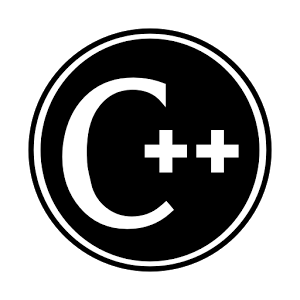# Local, Nested Class and ‘this’ pointer in C++

Local Class:-

• If a class is defined inside any function then that class is called as local class.
• This local class is used only inside a particular function.
• We can not create an object of this local class outside that function.
• According to above point local class works same as a local variable inside a function.
e.g.
class hello
{
void fun()
{
class demo
{
public:
int i;
};
demo d;
d.i=10;
} // function ends.
};
• In an above example, demo class is considered as a local class.
• In this example, object‘d’ of a class demo is destroyed after the execution of a function means the destructor of an object is called after exiting from a function.
• Using a local class concept we can give similar effect like namespace.

Nested class:-

• Nested class is similar as nested structure.
• In the nested class we have to define one class into some another class.
• In this case, the inner class can be accessible inside an outer class.
e.g.
class outer
{
public:
class inner
{
public:
int i;
};
private:
inner obj; // after this we can access an inner class.
};
• According to above, ‘obj’ is an object which is created inside an outer class and destroyed at the end of an outer class.

‘this’ pointer:-
e.g.
class demo
{
public:
int x,y,z;
int fun(int i)
{
cout<<i;
return 0;
}
};
int main()
{
demo d;
d.fun(10);
return 0;
}Explanation:-
• Every C++ program
is converted into C program and there are no concept objects in C.

• For this reason d.fun(10); is converted in some another form.
• In C++, when we call non-static member function then we use an object to call that function.
• A C++ compiler passes an address of that object as a first hidden argument in non-static member function.
• And this argument is accepted into a pointer and that pointer is of type class and this pointer is called as ‘this’ pointer.
• Syntax of ‘this’ pointer in our example is,
demo *const this;
• Expanded call of a fun() function is as follows d.fun(&d,10) is converted as
fun(&d,10);
• This first parameter is hidden from programmer but we can access this pointer from the function.
e.g.
class demo
{
public:
int x;
int fun(int i)
{
this->I;
this->y;
this->z;
(*this).x;
obj.x; // to access x;
}
};
• ‘this’ pointer is constant because of which we can not initialize an address of another object into ‘this’ pointer.
e.g.
this = &ob1j; // Error
• sizeof ‘this’ pointer is not included into the size of an object and ‘this’ pointer is visible from the function.
Use of ‘this’ pointer:-
1) we can access a members of the class as above.
2) We can write such a function which returns current object.
e.g.
demo fun(int i)
{
(this->x)++;
return *this;
}
• In this example, we change the value of x of caller object and that changed object is returned from the function by using ‘this’ pointer.
3) If the member arguments and arguments to the function are having same name, in that case we can use ‘this’ pointer to distinguish between them.
e.g.
int fun(int x,int y,int z)
{
this->x=x;
this->y=y;
this->z=z;
}

For more reading about technology news in singapore and seo to online marketing do view more about other pages.#### Sourabh Bhunje

Sourabh Bhunje, B.E. IT from Pune University. Currently Working at Techliebe. Professional Skills: Programming - Software & Mobile, Web & Graphic Design, Localization, Content Writing, Sub-Titling etc. http://techliebe.com/about-us